# SAT Math Multiple Choice Question 957: Answer and Explanation

### Test Information

Question: 957

9.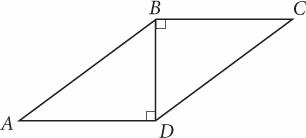Note: Figure not drawn to scale.

If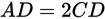and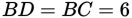, what is the length of side AB?

• A.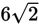• B. 12
• C.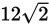• D. 18

Explanation:

D

Difficulty: Medium

Category: Additional Topics in Math / Geometry

Strategic Advice: Whenever you're given a figure, transfer as much information as possible from the question to the figure, and then label the figure with any additional calculations you make.

Getting to the Answer: Because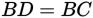, ΔBDC is an isosceles right triangle (and therefore a 45-45-90 triangle). You're told that the two legs both measure 6, which means that hypotenuse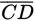measures. You also know that AD = 2CD, so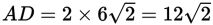. Next, use the Pythagorean theorem to find AB: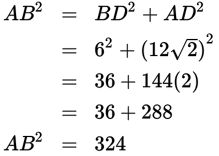So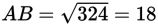, making (D) the correct answer.